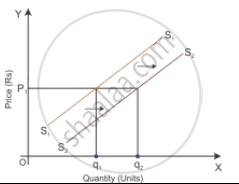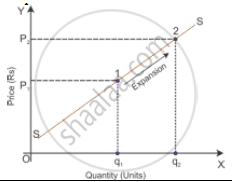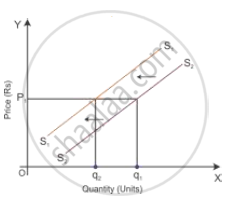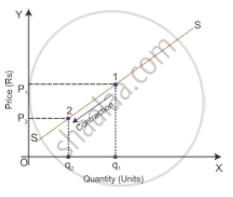Share

# Explain the Distinction Between “Change in Quantity Supplied’ and “Change in Supply”. Use Diagram. - CBSE (Arts) Class 12 - Economics

#### Question

Explain the distinction between “change in quantity supplied’ and “change in supply”. Use diagram.

#### Solution

 Basis Change in Supply Change in Quantity Supplied 1. Meaning Assume that the price remain constant, there is change in thesupply because of change in other variables such as technological change, input prices, unit tax and price of other related goods. Assume that other variables remain constant, there is achange quantity supplied because of the change in the own price of a good. 2. Functional relation Q_x=f(barP_X,P_Y,P_F,T,G,G_p,N_F)  where barP_X="Own price of a good" P_Y="Price of related goods" P_F="Price of factors of production" T="State of technology" G="Goal of the firm" G_p="Government policy" N_F="Number of firms" Q_x=f(P_x,barP_y,barT,bar(P_F),barG,barG_p,barN_F)  where barP_x="Own price of a good" barP_y="Price of related goods" bar(P_F)="Price of factors of production" barT="State of technology" barG="Goal of the firm" barG_p="Government policy" barN_F="Number of firms" 3. Shift and Movement The supply curve of the firm will shift rightward or leftward. The supply curve of the firm will move upwards or downwards. 4. Types Shift in the supply curve— increase in supply and decrease in supply. Movement along a supply curve—expansion of supply and contraction of supply. 5. Diagram for increase/expansion Increase in supplyExpansion in supply6. Diagram for decrease/contraction Decrease in supplyContraction in supplyIs there an error in this question or solution?

#### Video TutorialsVIEW ALL 

Solution Explain the Distinction Between “Change in Quantity Supplied’ and “Change in Supply”. Use Diagram. Concept: Supply Curve and Schedule.
S